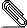# [NTG-context] equation refs broken?

Can someone confirm this? It seems that the equation reference mechanism is
broken some time in the recent past.
I have a small example done in
(1) ConTeXt  ver: 2011.07.13 20:14 MKIV
(2) ConTeXt  ver: 2011.11.17 23:31 MKIV
These are respectively the first and last pdf's below.


If true, it is very serious for me, because the I cannot prepare the upcoming
texts for my students!

Furthermore: \pagereference now introduces spurious white.

For the bad vertical spacing on the left I have submitted a tentative bugfix to
Hans Hagen.

Hans van der Meer

\setuppapersize[A6][A6]
\setupformulae[spacebefore=0pt,spaceafter=0pt]
\starttext
\placeformula[eq:one]\startformula a = 1 \stopformula
\placeformula[-]\startformula a = 2 \stopformula
\placeformula[eq:three]\startformula a = 3 \stopformula
\pagereference[ref:p]
Refer equation-1: \in{equ=<}[eq:one]> in page \at[ref:p].\par
Refer equation-3: \in{equ=<}[eq:three]> in page \at[ref:p].\par
\stoptextformularef.pdfformularef.pdf
___________________________________________________________________________________
___________________________________________________________________________________